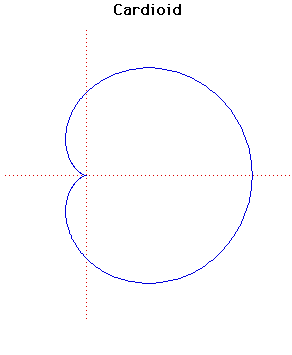# Curves

### CardioidCartesian equation:
$(x^{2} + y^{2} - 2ax)^{2} = 4a^{2}(x^{2} + y^{2})$
Polar equation:
$r = 2a(1 + \cos( \theta ))$

### Description

The cardioid, a name first used by de Castillon in a paper in the Philosophical Transactions of the Royal Societyin 1741, is a curve that is the locus of a point on the circumference of circle rolling round the circumference of a circle of equal radius. Of course the name means 'heart-shaped'.

Its length had been found by La Hire in 1708, and he therefore has some claim to be the discoverer of the curve. In the notation given above the length is $16a$. It is a special case of the Limacon of Pascal (Etienne Pascal) and so, in a sense, its study goes back long before Castillon or La Hire.

There are exactly three parallel tangents to the cardioid with any given gradient. Also the tangents at the ends of any chord through the cusp point are at right angles. The length of any chord through the cusp point is $4a$ and the area of the cardioid is $6\pi a^{2}$.

We can easily give parametric equations for the cardioid, namely
$x = a(2\cos(t) - \cos(2t)), y = a(2\sin(t) - \sin(2t))$.
The pedal curve of the cardioid, where the cusp point is the pedal point, is Cayley's Sextic.

If the cusp of the cardioid is taken as the centre of inversion, the cardioid inverts to a parabola.

The caustic of a cardioid, where the radiant point is taken to be the cusp, is a nephroid.

The cardioid is the catacaustic of a circle when the light rays come from a point on the circumference. This was shown by Jacob Bernoulli and Johann Bernoulli in 1692.

There are some other heart-shaped curves, sent to us by Kurt Eisemann (San Diego State University, USA):

(i) The curve with Cartesian equation: $y = 0.75 x^{2/3}$ ± $√(1 - x^{2})$. Show the picture

(ii) The curve with Polar equation: $r = \sin^{2}(\pi/8 - \theta/4)$. Show the picture

Other Web site:

Xah Lee

### Associated Curves

Definitions of the Associated curves